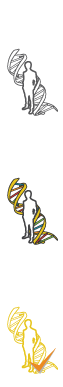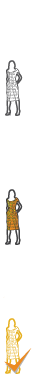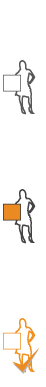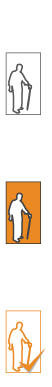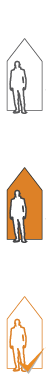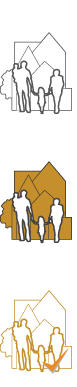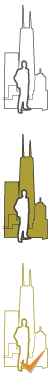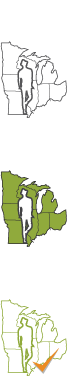#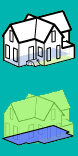Area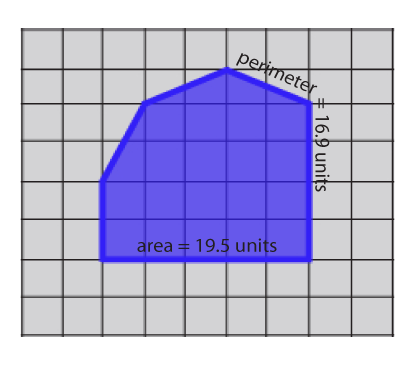Area and perimeter are two terms used to quantify distance and space. Often, there is confusion between the two. Perimeter is the distance around the outside of a closed area and is determined by adding all of the lengths of the object’s sides or its circumference. Area, in this usage, implies the total flat space that something takes up; area is the square foot or square metric measurement of the surface of a plane.

Exercises on 2D geometry introduce the concepts of flat space. Another way to think of area is to count how many units squares fits inside a figure, region, district, etc. A unit square is an area that measures one unit long by one unit wide. For example, It can be 1’ x 1’, 3.2m x 3.2m, 5yd x 5 yd, 1" x 1" or 10 miles x 10 miles! Area is everywhere…on every surface! You can measure an area of land, or the amount of area it takes to cover your house. You can even measure the area of skin on your hand. Simple equations are used to calculate area. A rectangle’s area can be found with height x width = area in square units. The area of a triangle is calculated as ½ base x height.

Be aware of area!

## Activity 1 – Find the Area of your Bedroom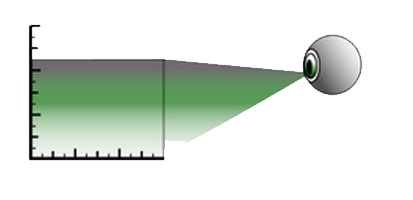Think about the area of your bedroom. What would be the best way to measure its area? Look at the Measurement journey exercises. This time, measure the length and the width of your room. It is easy to determine the area if you have a square room. Simply multiply the length of the room by the width of the room. Record your findings in your journal. Be sure to label the area with the squared measurement standard you used: feet, meter, centimeter or your hand.

## Activity 2 – Area is Everywhere!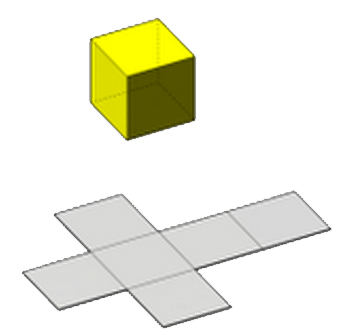Area is used to calculate many things. Roofers use area to determine how many bundles of shingles are needed to cover a roof. Architects use area to determine the number of floors in a building and how many square feet or square meters of usable space will be provided. Painters use area to understand how many gallons of paint will be needed. Farmers use area to determine how much seed to order for planting. Astronauts work with the surface area of planets.

Where do you use area? Think about art class - you have area in your sketch book to draw. Think about gym class - you have area in your gym to run. You already know how big your bedroom is, now measure the area of the walls. Measure the distance from the floor to the ceiling. A tape measure will work well. Finally, multiply this by the wall length you measured in activity 1 to get the surface area of your wall. Record your findings in your journal.

## Activity 3 – Determine the Area of your Home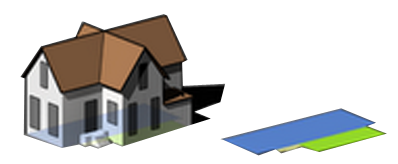Now take your new-found skill outside! Measure the area on the side of your house. If you are brave, measure the area of your home or building, you can then determine its footprint. This could be difficult if your home has many rooms! Try to break the footprint into measurable sections. Multiply the length and width of each section and add the areas of all of the rooms together to get the total footprint of your home!

## Activity 4 – Astounding Areas!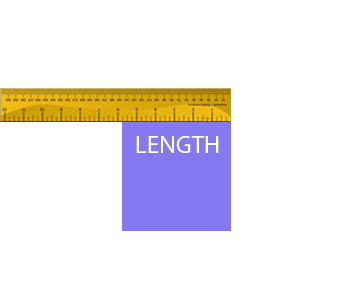Area is not like perimeter at all and is sometimes difficult to measure. Can you measure the area of one of your computer keys? Of the screen? Of the table or desk top? Of the floor of the room? Of the size of the building? Of the property the building sits on? Of the city the property is in? Of the county the city is in? Of the state the county is in? Of the country the state is in? Of the hemisphere the country is in? Of the world that has two hemispheres? Of the solar universe that holds the world? What is the nearest Area you can see? How large is the largest Area you can think of?

How will you begin to measure Area?

## Review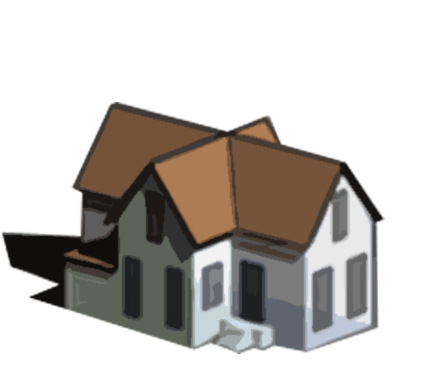• What is the formula for the area of a circle?
• Can you determine the surface area of a balloon before you blow it up?
• How many different surfaces are on a cube?
• How many different surfaces are on a sphere?
• Describe the difference between Area and Perimeter